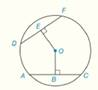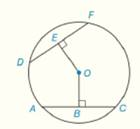Chapter 6.CR, Problem 26CR### Elementary Geometry for College St...

6th Edition
Daniel C. Alexander + 1 other
ISBN: 9781285195698

#### Solutions

Chapter
Section### Elementary Geometry for College St...

6th Edition
Daniel C. Alexander + 1 other
ISBN: 9781285195698
Textbook Problem
1 views

# Given :                               O E - ≅ O B -   i n   ⨀   O                                                       D F = x ( x - 2 )                                                                       A C = x + 28             F i n d :                                     D E   a n d   A CTo determine

To Find: DE and AC, If OE-OB- in  ODF=x(x-2) and AC=x+28Explanation

Concept:

Theorem 6.1.1:

A radius that is perpendicular to a chord bisects the chord.

Theorem 6.1.7:

Chords that are located at the same distance from the center of a circle are congruent.

Theorem 6.1.8:

Congruent chords are located at the same distance from the center of a circle.

Calculation:

Given that OE-OB- in  O

So, OE and OB are congruent distances.

So, by Theorem 6.1.7:

Chords that are located at the same distance from the center of a circle are congruent.

DF and AC are at the same distance from the center of a circle  O.

So, DF=AC

But it is given that, DF=x(x-2) and AC=x+28

So, x(x-2)=x+28

By distributive property, x2-2x=x+28

Subtract x+28 from each side

x2-2x-x-28=x+28-x-28

x2-3x-28=0

Factorize, x2-7x+4x-28=0

xx-7+4

### Still sussing out bartleby?

Check out a sample textbook solution.

See a sample solution

#### The Solution to Your Study Problems

Bartleby provides explanations to thousands of textbook problems written by our experts, many with advanced degrees!

Get Started